# LINEAR Axes

## Setting the Axis Data Range and Tick Values

For a LINEAR axis, you can set the tick values in several ways. If you use TICKVALUELIST = ( values ) or TICKVALUESEQUENCE= ( start-end increment ) syntax, the values that you specify will be used as long as those values are within the actual range of the data. Notice in the following example that the smallest and largest tick values on the Y axis are not what was requested because the Y-axis data range did not include 0 or 8000000. To extend (or reduce) the axis data range, you can use the VIEWMIN= and VIEWMAX= sub-options of the LINEAROPTS= option. Notice that because the X-axis was extended with these options, all the specified tick values were used. The X-axis also illustrates that the tick values do not have to be uniformly spaced. (Please note that choosing tick values in this manner does NOT create a log scale. See LOG Axes for information about log axes.)
```layout overlay /
xaxisopts=(linearopts=( viewmin=0 viewmax=16
tickvaluelist=(0 2 4 8 16)  ))
yaxisopts=( linearopts=
( tickvaluesequence=(start=0 end=8e6 increment=1e6) ));
seriesplot x=Hours y=Bacteria;

endlayout;
```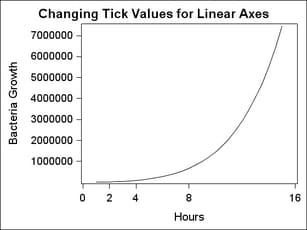## Formatting Axis Tick Values

Linear axes use special techniques that provide the generation of "good" tick values that are based on the data range. If a tick value format is not specified, the column formats provide a "hint" on how to represent the tick values, but those formats do not generally control the representation or precision of the tick values.
To force a given format to be used for a linear axis, you can use syntax similar to the following, where you specify any SAS numeric format:
`	linearopts=(tickvalueFormat= best6. )`
Note: GTL currently honors most but not every SAS format. For a list of supported formats, .
If you simply want the column format of the input data column to be directly used, specify the following:
`	linearopts=(tickvalueFormat=data)`
There are special options to control tick values. INTEGER=TRUE calculates good integers to use as tick values given the range of the data. EXTRACTSCALE=TRUE can be used to extract some factor of ten from all tick values in order to reduce the overall width of the tick values and improve legibility. The extracted factor is concatenated to the existing axis label. In the following example, a factor of 1000000 (million) is extracted from the Y-axis values and the text (million) is appended to the axis label.
```layout overlay /  xaxisopts=(linearopts=(integer=true))
yaxisopts=(linearopts=(tickvalueFormat= (extractScale=true) ));
seriesplot x=Hours y=Bacteria;
endlayout;
```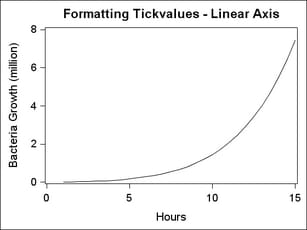## Avoiding Tick Value Collisions

Another intelligent feature that axes have is to change the display of tick values whenever the tick value text becomes too crowded. For example, the axis below comfortably shows eleven tick values: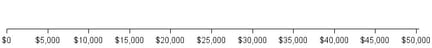If the size of the graph decreases or the font size for the tick values increases, the axis ticks and tick values will automatically be "thinned" by removing alternating ticks and tick values. LINEAROPTS = (TICKVALUEFITPOLICY=THIN) is the default action for linear axes: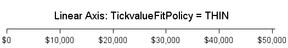You can set TICKVALUEFITPOLICY=ROTATE which angles the tick value text 45 degrees: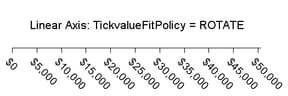You can set TICKVALUEFITPOLICY=STAGGER which creates alternating tick values on two rows.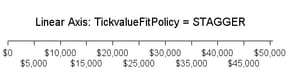You can set TICKVALUEFITPOLICY to a compound policy ROTATETHIN, STAGGERTHIN, or STAGGERROTATE. The compound policies attempt the second policy if the first policy does not work well. These policies are available for X and X2 axes. The only fit policy for the Y and Y2 axes is THIN.
Note: The TICKVALUEFITPOLICY= is never applied unless a tick value collision situation is present. That is, you cannot force tick values to be rotated or staggered if there is no collision situation.